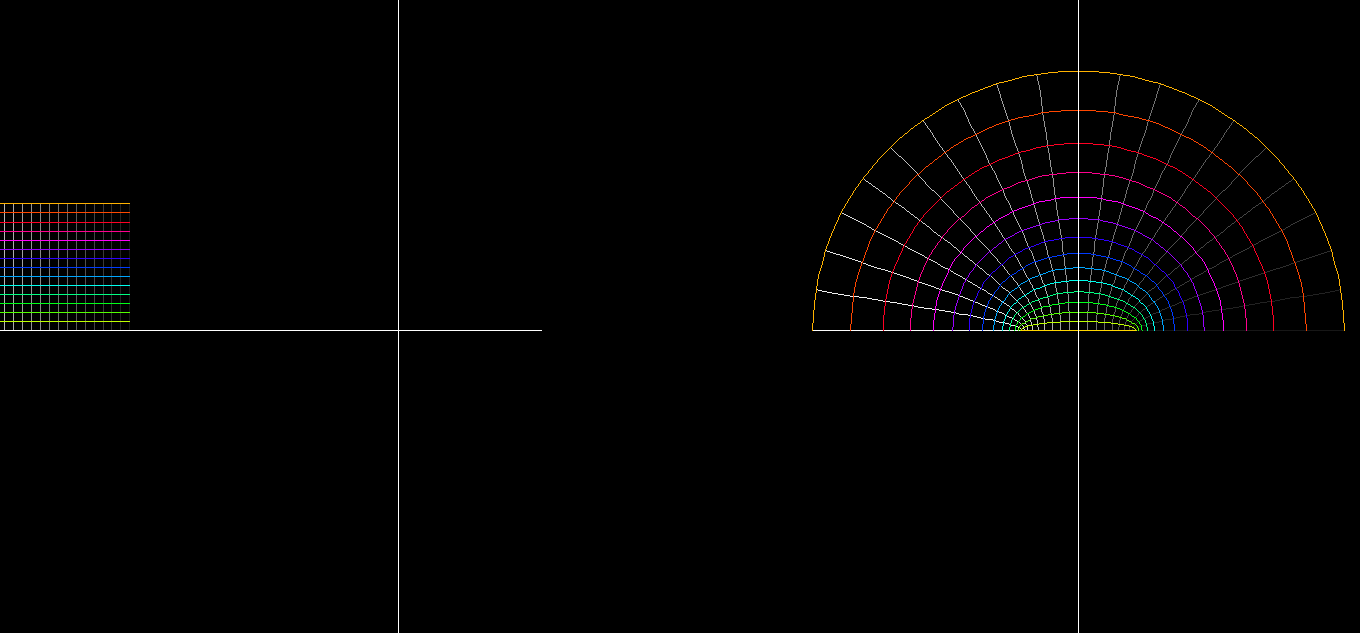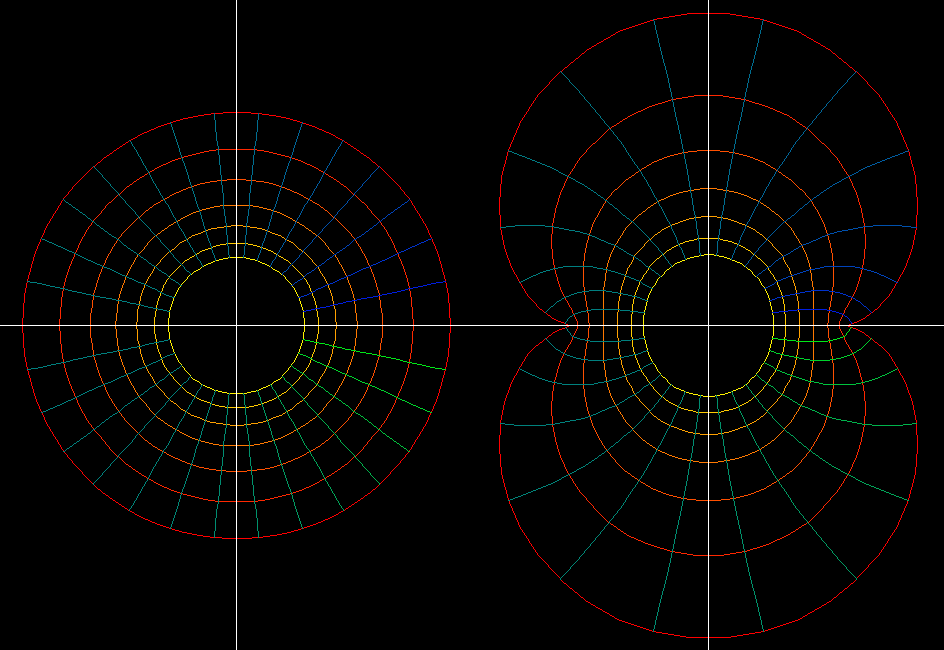# Complex Sine Function

The complex sine function is, as in the real case, defined as the solution of the differential equation (ODE) `sin''(z) = -sin(z)` to the initial conditions `sin(0) = 0, sin'(0) = 1`. The real and the complex sine function therefore agree for real arguments x. The function `g(z) = sin(i*z)` satisfies the differential equation `g''(z) = +g(z) with g(0) = 1, g'(0) = i`. By definition of the hyperbolic sine as solution of this ODE we conclude `sin(i*z) = i*sinh(z)`.function: z → f(z) = sin(c*z)/c domain: rectangular grid: -1.57 ≦ Re(z) ≦ 1.57; -2 ≦ Im(z) ≦ 2 morph : 1 ≥ c ≥ 0: as c approaches 0 the function converges to the linear function z → z. The ODEs imply: exp(z) = cosh(z) + i*sinh(z) = cos(i*z) + sin(i*z), and also cos(z) = sin(z + pi/2). Therefore one can read the values of sin, cos, sinh, cosh from this one picture - in the same way as the graph of the real sine function also gives the values of the real cosine function. As with all conformal maps f: the parameter rectangles in the range get very small with strongly curved edges near values f(z) where f'(z) = 0. Of course sin'(pi/2) = 0 = sin'(-pi/2) as in the real case.function: z → f(z) = sin(c*z)/c Preimage: rectangular grid in domain: -pi/2 + b ≦ Re(z) ≦ pi/2 + b ; 0 ≦ Im(z) ≦ 2.5 morph: -2*pi ≦ b ≦ 2*pi. This morph shows the periodicity of the complex sine function, sin(z) = sin(z+2pi). Since sin(z) grows fast for z = i*y on the imaginary axis (recall sin(i*y) = i*sinh(y)) we can draw only a small portion of the fundamental domain {z ; -pi ≦ Re(z) ≦ pi} for the period translations.function: z → f(z) = sin(c*z)/c domain: polar grid 0.5 ≦ |z| ≦ pi/2, 0 ≦ angle ≦ 2*pi morph : 1 >= c >= 0.

z_sin.pdf